#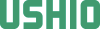Journal of Physics D : Applied Physics 38号（2005年）

# In situ measurements of electrode work functions in free-burning arcs during operation at atmospheric pressure

Manabu Tanaka 1 , Masao Ushio 1 , Mitsuru Ikeuchi 2 and
Yoshiro Kagebayashi 2
1 Joining and Welding Research Institute, Osaka University, 11-1 Mihogaoka, Ibaraki, Osaka 576-0047, Japan
2 Lamp Technology & Engineering Division, USHIO INC., 1194 Sazuchi, Bessho-cho, Himeji, Hyogo 671-0224, Japan
Received 15 August 2004, in final form 13 October 2004
Published 16 December 2004
Online at stacks.iop.org/JPhysD/38/29

## Abstract

A method of in situ measurement of electrode work functions in free-burning argon arcs during operation is presented. This technique is based on the photoelectric effect ocurring at the surface of a tungsten cathode with the use of a pulse laser system consisting of a NdーYAG laser and a dye laser. Three types of tungsten electrode, namely, pure W, Wー2% ThO2 and Wー2% La2O3 are used in this work. Free-burning arcs are operated in argon at atmospheric pressure at currents of 100 A and 200 A.The effective work functions of pure W, Wー2% ThO2 and Wー2% La2O3 electrodes during operation at a current of 200 A are found to be, respectively, 4.6, 2.8 and 3.0 eV from in situ measurements. These results are very close to the work functions of pure W, ThO2 and La2O3 obtained from the literature. The in situ measurements, however, show that the effective work functions of pure W, Wー2% ThO2 and Wー2% La2O3 electrodes for an 100 A arc are, respectively, 2.9 eV, 2.6 eV and 2.0 eV. It is shown that each effective work function for an 100 A arc clearly becomes lower than that for a 200 A arc for all types of tungsten electrodes.
(Some figures in this article are in colour only in the electronic version)

## 1. Introduction

Tungsten is the most commonly used electrode material for electric arcs because of its high melting point and high boiling point. There are many applications of electric arcs in industrial processing of materials, for example in welding, cutting, thermal spraying, heating, surface treatment and chemical vapour deposition (CVD) [1, 2]. In most electric arcs, especially in freeburning arcs at atmospheric pressure where the arc current is emitted from a tungsten cathode electrode, the work function should be reduced, generally by adding an emitter material such as thorium oxide (ThO2) or lanthanum oxide (La2O3) [3, 4]. The current flow from the cathode of a free-burning high-intensity arc at atmospheric pressure is characterized by a‘diffuse mode‘and thermionic emission of electrons is the governing mechanism for liberation of electrons from the cathode in this mode . In general, thermionic electron emission from the cathode surface is a function of the cathode temperature and of the work function of the electrode material, which is today known as the RichardsonーDushman equation . Since thermionic emission requires a very high surface temperature close to the melting point of tungsten (3653 K), energy transfer from the free-burning arc to the cathode electrode is necessary for maintaining this high surface temperature. For example, ions accelerated by the field in the cathode fall zone of the arc plasma heat the cathode surface by impinging on it and also give energy for recombination with electrons at the cathode surface. Furthermore, an arc plasma reaches a temperature of 17000 K  and heats the cathode surface by thermal conduction .

However, there is still a lack of practical understanding of the physical behaviour in the electrode region. For example, there is a potential drop which corresponds to extremely steep gradients of the space potential in front of the cathode electrode, namely, the cathode fall, but the actual state of the potential drop or the electric field strength has not been demonstrated experimentally [8, 9]. The work functions of pure materials such as W, ThO2 and La2O3 are well known from [10, 11]. But the work function or effective work function of a tungsten electrode in free-burning arc during operation has not been made clear, although the cathode fall would affect the electron emission from the cathode surface, which is suitable for the field emission . Measuring the effective work function of tungsten electrodes during operation would be extremely helpful for a better understanding of the physical behaviour in the electrode region of free-burning arc at atmospheric pressure, which would contribute to developments of advanced control and evaluation systems for materials processing by the arcs through the predictions of properties of the total arc processing system, for example, using a unified calculation of the tungsten cathode, arc plasma and anode material [12, 13].

The previous landmark investigation of the work function of tungsten electrodes during operation was made by Schlagerand Neiger . They carried out in situ measurements of electrode work functions for a HID (high-intensity discharge)lamp by using the pulse laser system and showed the effective electrode work functions of tungsten electrodes during operation. However, HID lamps are generally operated in a bulb filled with high-pressure gases up to 50 bar and at lower arc currents up to 10 A . They employed HID high-pressure lamps operated at 50 Hz cycle frequency in quartztubes filled with mercury, although quantitative values about lamp currents and operating pressure were not explained. Therefore, no information on the effective work function of tungsten electrodes was given for free burning high-intensity arcs at atmospheric pressure by their investigation.

This paper is concerned with in situ measurements of electrode work functions in the free-burning arc during operation at atmospheric pressure. This technique is based on the photoelectric effect at the surface of tungsten cathodes by using the pulse laser system . We present experimental results of work functions for three types of tungsten electrode, namely, pure W, Wー2% ThO2 and Wー2% La2O3 during operations at 100 and 200 A in arc currents, in comparison with the work functions of W, ThO2 and La2O3 as pure materials from [10, 11].

## 2. Experimental method

The experimental method is that of Schlager and Neiger, which is based on using 'Fowler plots'  for estimating the work functions of materials. The tip of a tungsten electrode used as the cathode of a freeburning arc was irradiated by laser pulses. The pulse laser system consisted of a NdーYAG laser (model SL800, Spectron Laser Systems) and a dye laser(model SL4000G, Spectron Laser Systems). Figure 1 shows a schematic drawing of a system of experiments for in situ measurements of electrode work functions. The Nd ーYAG laser simultaneously generated the fundamental wavelength(ω) and the second harmonic (2ω) with a pulse duration of 15 ns and a pulse repetition rate of 10 Hz. The second harmonic(2ω) was pumped into the dye laser and then generated the fundamental wavelength (ωdye) and the second harmonic(2ωdye) of the dye laser. The wavelength generator and separator (model SL4000D-FMX-A, Spectron Laser Systems) in figure 1 was able to produce laser pulses at wavelengths of 1064, 640, 532, 400, 385, 320, 300 and 237 nm by mixing ωdye or (2ωdye) with or withoutω. Table 1 shows the wavelengths used in this work and their related photon energies. The second column shows every source of laser wavelength which is obtained by mixing the dye laser with or without the NdーYAG laser. The first column shows the types of dye, but no dye is used for the two wavelengths of 532 nm and 1064 nm, obtained from the fundamental wavelength (ω) and the second harmonic (2ω) of the NdーYAG laser, respectively. The laser light was focused on the tip of the tungsten electrode using an optical lens of focal length 600 mm. The pulse energy of the laser was regulated to be about 1 mJ independent of the wavelength for avoiding local thermal heating of the electrode by the absorbed laser energy. We have measured the effective photoelectric yield, Y , defined as the ratio of the number of emitted photoelectrons, Ne, to the number of photons absorbed into the electrode, Np. This ratio, Y , is represented as

where jph is the electron current due to photoelectric emission, e is the elementary charge, Ilaser is the laser intensity absorbed into the electrode, h is Planck‘s constant, ν is the laser frequency, R is the measuring resistance, U is the voltage concerned with the photoelectric signal at the measuring resistance, R ,Δt is the pulse duration of the laser, E pulse is the pulse energy of the laser and ε is the electrode absorption, obtained from .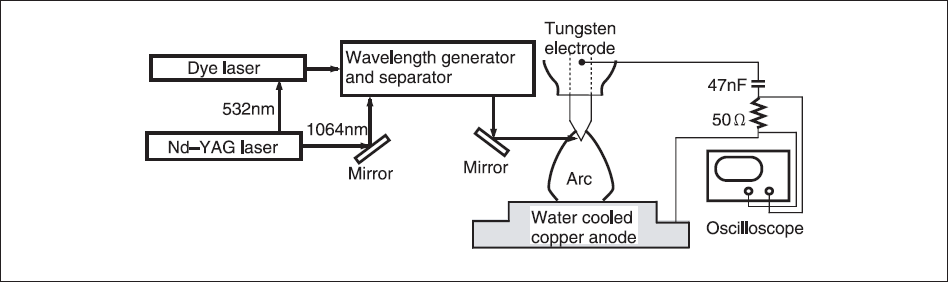Figure 1. Schematic drawing of a system of experiments for in situ measurements of electrode work functions.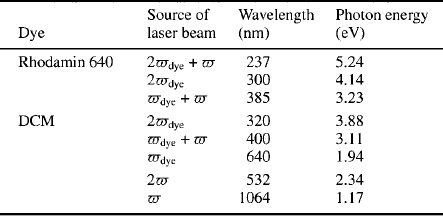Table 1. Wavelengths of the lasers used in this work and their related photon energies.

To detect the photoelectric signal arising from the pulse irradiation of the electrode, a resistanceーcapacitor combination(R=50ω, C=47 nF) was used in parallel to the free-burning arc, as shown in figure 1 . The short-duration signal of voltage U at the resistance R was measured using a fast digital oscilloscope (model TDS3052, Tektronix, maximum 5 GHz). This measurement involved integration of 128 laser pulses. Since the photoelectric yield, Y , represented by equation (1) was, therefore, measured experimentally, the effective work function during operation should be estimated by fitting with Fowler‘s theoretical function , given by

where A is a constant, kB is the Boltzmann constant, T is the temperature and Φ is the work function. Equation (2) clearly shows that the photoelectric emission is a function of the temperature, the work function of the material and the photon energy. If the surface temperature of the electrode is measured experimentally, equation (2) gives us the effective work function,Φ, by being fitted to the experimental plot data in equation (1).

Continuous-wavelength pyrometry was used to measure the surface temperature of the tungsten electrode to avoid problems arising from the emissivity of the tungsten electrode, which will be dependent on the emitter materials. This technique is based on multiwavelength pyrometry and obtains the temperature from Planck‘s formula by fitting to experimental data. Measurements of the continuous spectrum from 612 to 614 nm at the tip of tungsten electrode were carried out using an optical system consisting of optical lenses, an optical fibre, a monochromator (model HR-1000, Jobin Yvon) and an ICCD detector (InstaSpec V, ORIEL Inst.) as shown in figure 2. Measurements were made at times 2 ms after switching off the arc by simultaneously using a pulse generator (model DG535, Stanford Research Systems) to avoid the noise problems arising from the intensive radiation of the arc. Haidar and Farmer  showed that experimental values of the tip temperature measured at less than 5 ms after switching off the arc were in agreement with tip temperatures measured during operation. The measurement after switching off the arc was by a simple method and was also easier than the measurement during operation, for which it was necessary to employ special techniques used for estimates of plasma radiation . To understand the behaviour at the tip of the tungsten electrodes during operation, a digital video (Phantom V, Vision Research) was used for observations as shown in figure 2.

The free-burning arcs were generated between a tungsten electrode and a water-cooled copper anode (diameter 50 mm) in argon at atmospheric pressure, as shown in figure 3. The arc gap, namely, the cathode-to-anode gap, was fixed at 5 mm and the argon gas was fed from a torch nozzle with a flow rate of 15 litre min－1 . In order to avoid the electrical noise and the radio noise arising from the inverter-controlled welding power source, a lead storage battery (model SBW170D, Shindaiwa Kogyo) was used so that the signals from the photoelectric effect could be detected. The arc was first generated by using an inverter-controlled power source, and then a switchover between this power source and the lead storage battery was carried out, except when measuring the tip temperature of the tungsten electrode, because of the need to control simultaneously for switching off the arc as shown in figure 2. Free-burning argon arcs were operated at currents of 100 A and 200 A. Three types of tungsten electrode, namely, pure W, Wー2% ThO2 and Wー2% La2O3 were tested in this work. All types of tungsten electrode, of 3.2 mm diameter, were initially shaped to a cone with a 60° included angle.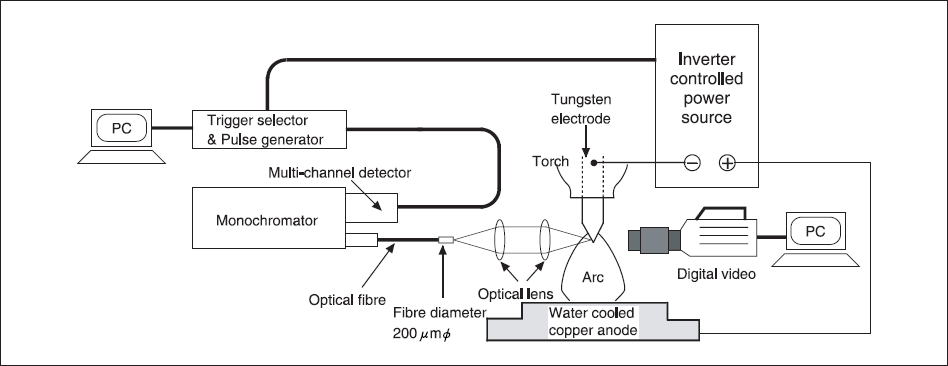Figure 2. Schematic drawing of a system of experiments for temperature measurements and observations of a tungsten electrode tip.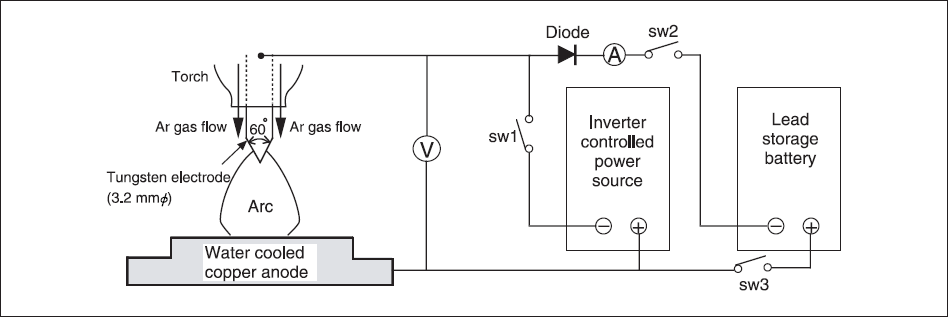Figure 3. Schematic drawing of a system of experiments for generation of free-burning argon arcs at atmospheric pressure.

## 3. Results and discussion

Table 2 shows results of measurements of the temperature of the tip of the tungsten electrode during operation using the continuous-wavelength pyrometry technique. Each temperature is the average of ten measurements. The result obtained from the averaged value varied a little, and its error was about ±10%. The temperatures in the case of 200 A are higher than the temperatures for 100 A for all types of electrode. The temperatures for pure W electrodes are obviously higher than the melting point of tungsten (3653 K) for both arc currents, and the molten tips of the electrodes were clearly observed using the digital video during operation, as shown in figure 4. Figure 4 shows photographs of typical behaviour at the tip of the tungsten electrodes during operation for a 200 Aarc and also the appearance of the tip after operation for 60s. The temperatures of the Wー2% ThO2 electrode are slightly higher than the tungsten melting point. Traces of the molten metal were observed at the tips after switching off the arcs, as shown in figure 4, for both arc currents. These results are in good agreement with previous experimental results [17ー19] and have been given in equation (2) for fitting with the experimental plot data related to equation (1).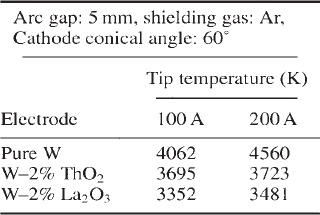Table 2. Experimental values of the temperature of the tip of the tungsten electrode during operation of a free-burning argon arc obtained using the continuous-wavelength pyrometry technique.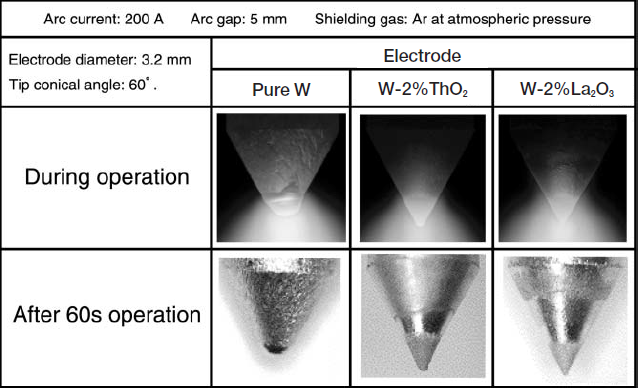Figure 4. Photographs of typical behaviour at the tip of tungsten electrodes during operation for a 200 A arc and also appearances of the tip after operation for 60 s.

Figure 5 shows a typical photoelectric signal for the Wー2% ThO2 electrode at an arc current of 200 A. This short-duration signal was detected at the resistance R as shown in figure 1. Figure 6 shows the photoelectric yields, Y , against photon energies for (a) pure W, (b) Wー2% ThO2 and (c) Wー2% La2O3 at an arc current of 200 A. Each experimental plot in figure 6 is the average of three measurements. The result obtained from the averaged value varies by ±15% at the maximum, which appears on each error bar in figure 6. Every solid curve represents a fit with Fowler‘s theoretical function of equation (2), and the effective work function is determined from this fit. Since the work function appears inside an exponential plot, the effect of the above experimental errors on the derived effective work functions is small. The errors, however, arose from the experimental errors in the temperature measurements of the tungsten electrode together with the above-mentioned experimental plot errors in figure 6. The errors were about ±5% in the derived effective work functions.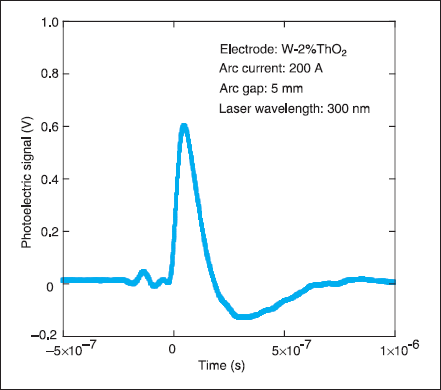Figure 5. A typical photoelectric signal for a Wー2% ThO2 electrode at an arc current of 200 A.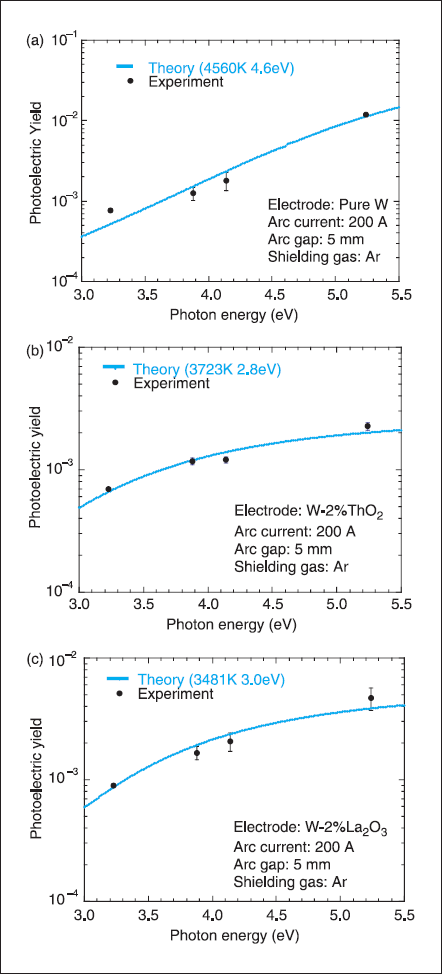Figure 6. Photoelectric yields against photon energies for (a) pure W, (b) Wー2% ThO2 and (c) Wー2% La2O3 at an arc current of 200 A. Every solid curve represents a fit with Fowler's theoretical function, and the effective work function is determined from this fit.

Table 3 shows experimental values of the effective work function of the tungsten electrode during operation at arc currents of 200 and 100 A . Table 3 also shows the work functions of pure W, ThO2 and La2O3 from [10, 11]. The values in square brackets give the range of scattered data for each material, and then the work functions from the literature are given by their average values. Table 3 shows that the effective work functions of pure W, Wー2% ThO2 and Wー2% La2O3 electrodes during operation at a current of 200 A are very close to the work functions of W, ThO2 and La2O3 as pure materials, respectively. It is suggested that the effective work function of a tungsten electrode with an added emitter material is dominated by the work function of the emitter material, even though the addition to the electrode is only 2% in weight. Figure 4 shows that the molten tips of the electrode are clearly seen for pure W and the rounded shape appeared a few seconds after switching on the arc. However, the electrode tips remained conical during operation for Wー2% ThO2 and Wー2% La2O3. This difference is primarily caused by the difference in the effective work functions of the tungsten electrode. The fraction of the ion current to the total current is in the range from 15% to 50% at the cathode surface, which is dependent on the arc discharge conditions related to the arc current, electrode material and shielding gas . The ions heat the tungsten electrode by recombination with electrons at the cathode surface . Even though the higher work function of the pure W electrode would reduce the thermionic electron emission from the cathode surface for an instant after arc ignition, the high arc current of 200 A is able to produce a sufficient ion flux towards the cathode, namely, the ion current. This intensive heating due to the recombination of ions with electrons would melt the tip of the pure W electrode for a few seconds after arc ignition as shown in figure 4 and then sufficient thermionic electron emission from the cathode surface would arise. This balance of the ion current and the electron current would not change the effective work function of the pure W electrode in comparison with the work function of W. Since the lower work function of the electrode for Wー2% ThO2 and Wー2% La2O3 would produce sufficient thermionic electron emission from the cathode surface, the fraction of the ion current to the total current will be small and then the temperature of the tip of the electrode will remain low.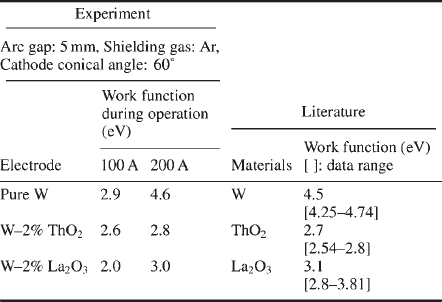Table 3. Experimental results of the effective work function of a tungsten electrode during operation of a free-burning argon arc at arc currents of 100 A and 200 A and the work functions of pure W, ThO2 and La2O3 from [10, 11].

Furthermore, table 3 shows that each effective work function during operation for the 100 A arc is clearly lower than that for the 200 A arc. It is suggested that the effect of the extremely large gradients of the space potential in front of the cathode electrode, which is a well known characteristic phenomenon at the cathode, is to lower the work function. Since a lower arc current than 200 A is not able to produce sufficient ion flux towards the cathode, the lower thermionic electron emission from the cathode surface, arising from the lower temperature of the tip of the electrode, increases the fraction of the ion current to the total current at the cathode surface. This is consistent with the formation of a positive space charge which determines the cathode fall zone . The strong positive space charge reduces the effective electrode work function due to the Schottky Schottky effect . Schlager and Neiger  showed that the effective electrode work function for the spot attachment mode at the cathode surface for high-intensity discharge lamps was clearly lower than that for the diffuse attachment mode owing to the Schottky effect. They thought that a switchover between the diffuse and spot modes could be forced by reducing the lamp current. However, they did not provide quantitative values for the lamp currents and operating pressure. Furthermore, they did not measure the temperature of the tip of the tungsten electrode but assumed the temperature to be 2000 or 3000 K, independent of the attachment mode at the cathode surface. The current flow from the cathode of a free-burning high-intensity arc at atmospheric pressure is characterized by a ‘diffuse mode‘ . Our observations using the digital video during operation showed the diffuse mode in the cases of both 100 and 200 A arc current. Pfender expounded the idea that an arc in diffuse mode displays an appreciable constriction in front of the cathode so that the actual current transition zone appears as a ‘spot‘ . He also expounded the idea that the cathode attachment in the diffuse mode was stationary, in contrast to the spot mode which frequently showed one or several spots moving with high velocities and randomly over the cathode surface . Schlager and Neiger  did not explain in detail the difference between their diffuse and spot modes. They simply explained that a transition from the diffuse mode to the spot mode could be made by reducing the lamp current.

The reduction in the work function arising from the Schottky effect is represented by

where ΔΦis the reduction in work function (eV) and E is the electric field strength (V cmー1 ) . Using equation (3) and the reduction in the effective work function during operation from the 200 A arc to the 100 A arc in table 3, we can obtain a rough estimate of the average electric field strength in the cathode fall for a current of 100 A. We obtain 20.1 ×10 6 Vcmー1 for a pure W electrode, 0.3 ×10 6 Vcmー1 for a Wー2% ThO2 electrode and 7.0 ×10 6 Vcmー1 for a Wー2% La2O3 electrode. These estimations help us to understand the physical behaviour in the cathode region, characterized by an intense electric field in the cathode fall of a free-burning arc at atmospheric pressure.

## 4. Conclusions

The conclusions of this work are summarized as follows.
• (1) A method of measurement in situ of the electrode work function in a free-burning arc during operation at atmospheric pressure in argon has been demon-strated. The effective work functions of pure W, W ー2% ThO2 and Wー2% La2O3 electrodes during operation at a current of 200 A were, respectively, 4.6, 2.8 and 3.0 eV from the in situ measurements. These results were very close to the work functions of pure W, ThO2 and La2O3 obtained from the literature.
• (2) The effective work function of tungsten electrodes with an added emitter material, namely ThO2 or La2O3, was dominated by the work function of the emitter material, even though its addition to the electrode was only 2% by weight.
• (3) Each effective work function during operation for a 100 A arc was clearly lower than that for a 200 A arc for all types of tungsten electrode. Those of pure W, Wー2% ThO2 and Wー2% La2O3 electrodes for an 100 A arc were, respectively, 2.9 eV, 2.6 eV and 2.0 eV. Our opinion about the possibility of the reduction in the effective work function is that the lower thermionic electron emission from the cathode surface arising from the lower temperature of the tip of the electrode reduced the effective electrode work function owing to the Schottky effect.

## Acknowledgments

We would like to thank Dr J J Lowke in CSIRO, Sydney, Australia, for many important suggestions and for a critical reading of the manuscript.